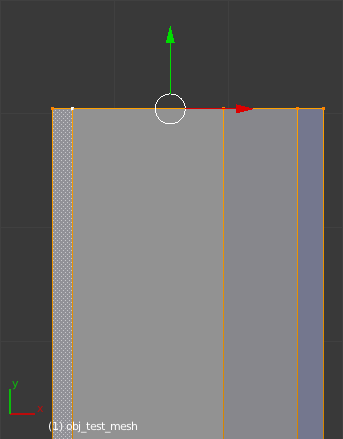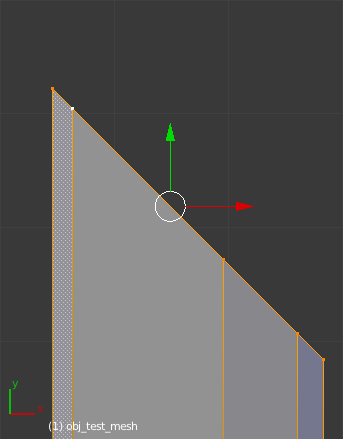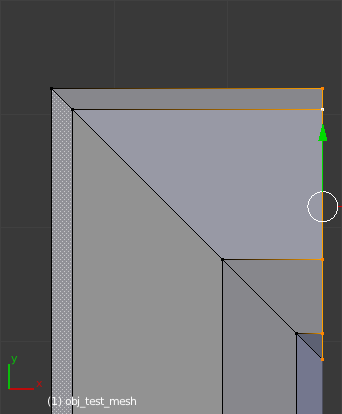# Square Spin?

Is there any existing scripts that can do this? If not, any recommended methods to go about doing it in 2.63+ python.Ideally I would want to make it spin clockwise or counterclockwise based on the view and the last selected vertex would be the pivot. I wrote a script that kinda does this but is fixed to each axis (Square Spin Z, Square Spin Z CC, Square Spin X, etc).

would like to have one like that !

that would be useful and save time when doing moldings ectl

anyone can try to make a little script for this one function

thanks
happy blendering

Well I have a script that does it as a local coord axis-aligned extrude. I suppose I could clean it up a bit and post it as an add-on. What I would really like to do is make it’s controls automatic based on the aligned views (Top, Bottom, Front, etc.).

it would be cool

there shoudl be a way to detect if a window is front back ect…
but don’t remember how to !

thanks

SynaGl0w: check out the LoopTools addon
http://wiki.blender.org/index.php/Extensions:2.6/Py/Scripts/Modeling/LoopTools

Flatten with Plane: View flattens based on current view direction, maybe that helps

The “Extrude along edge path or loop” tool is nice, but I am working on something for doing fast square corners without relying on a separate edge, loop, or curve. Just what you have selected at the time.

I am working on making the controls 100% manual and 100% auto.

There will be buttons for up and down, left and right, or sometimes both, in the tool shelf that may be pressed to extrude a square corner from whatever is selected. Again, no edges, loops, or curves needed.

Python is not really my thing (I’m more of a C++ guy). Anyway I am making the operators go off what the view-port angle is relative to the axes of the object and the direction(sometimes normal) of the selection. I decided I wanted to keep the corners axis aligned so they should remain pretty accurate. Most of the time I don’t need to make such a corner at an odd angle and if I do I’ll use some of the much more manual methods.

Did a quick test of my AxisAlignment class which will dictate the operators settings.

This way the view-port does not need to be aligned perfectly with what you are working on and controls will work as expected.

would be nice to be able to select different shape
like rounded corner or squarish or ellipse et…

salutations

ok – my idea … because i stumpled over this kind for my grid-snaping problem.

you have some vertices adjusted on a plane (normaly the end of some mesh-extrusion like cut with a knife) and now you want to spin this part around the (lets say) right (like in your picture) vertex.

(from looking at some kind of grid-view setup from these vertices, its not an even spaced grid)
calculation could do:
first step is the (virtuell, math-calculation) duplication of the vertices and rotation with 90 degrees for the position of the end-plane. The rotation is done around the (in the above sample) right view-axis.
then for each corner vertices the position could be calculated from end-position subtracting the vector, that is the (shortest) difference from the starting-point to its spin-axis.

I dont know if its easy to use 2 extrusions, where the second extrusion is rotated around 90 degrees and the calculation is used to set the new locations for the vertices of the first extrusion ( and later do a remove-duplicated vertices to get rid of the not to be extruded vertices).

wanted to do a first try … its for blender-2.61 (thats what i still use most times)

the script is in the blend-file for rotation around the x-axis.

like the hint-text in the blend-files says:

run the script (of the text-window above the 3dview)
switch to edit mode (and the cube should show the upper row of vertices selected)
press space-bar
and select the
test spin
function to to a basic rectangle/quat-like rotation.

what is missing is adjustment for different rotation axis and
if this works the removing of duplicate-vertices of the 2-step extrusion.

``````
# test-dr / 12-06-05
# testing some kind of square-90-degrees-rotation
# with the help of the rotate-function

import bpy
from math import sqrt

#try to calculate the rotation-point and
# and a first scale along this vector to move to square-position
#    print(lp1, lp2, co)
x1,y1,z1 = lp1 # first point of rotation-line
x2,y2,z2 = lp2 # axis/direction of line from first point
#caluclate second point of line for line-equation like: p = p1 + u(p2-p1)
x2 += x1
y2 += y1
z2 += z1
x3,y3,z3 = co # the rotated point-coords, calc nearest point on rotation-axis
# u = position on line according to line-equation
u = (x3-x1)*(x2-x1)+(y3-y1)*(y2-y1)+(z3-z1)*(z2-z1)
#    print("u:",u)
# calculate point on rotation-axis
x0 = x1 + u * x2
y0 = y1 + u * y2
z0 = z1 + u * z2
#    print("intersection:", x0,y0,z0)
# calculate vector to do the scaling along
xn =  (co - x0)
yn =  (co - y0)
zn =  (co - z0)
# my .. arghh .. rounded scale-factor
sc = 0.82 / sqrt( xn*xn + yn*yn + zn*zn )
return([sc*xn + co, sc*yn + co, sc*zn + co])

def main(context):
ob = context.active_object
if ob:
print(ob.name)
if ob.type == "MESH":
mesh = ob.data
nv = len(mesh.vertices)
print(nv, "no. vertices")
center = (0,0,0)
axis   = (1,0,0)
bpy.ops.object.mode_set(mode="OBJECT")
vselect=[] #do i need the old selected vertices?
for v in mesh.vertices:
if v.select:
vselect.append(v.index)
bpy.ops.object.mode_set(mode="EDIT")
bpy.ops.mesh.spin(steps=2, dupli=False, degrees=90, center=center, axis=axis)
#mesh.update()
bpy.ops.object.mode_set(mode="OBJECT")
bpy.ops.object.mode_set(mode="EDIT")
nv2 = len(mesh.vertices)
print(nv2, "no. vertices new")
bpy.ops.object.mode_set(mode="OBJECT")
for i in range(nv, nv2):
if i &lt; nv+ (nv2-nv)/2:
#print(i, mesh.vertices[i].co[:])
if mesh.vertices[i].co &gt; 1.1: #not on rot-axis
#try adjust like scaling relativ to rot-axis
print(i, mesh.vertices[i].co[:])
i2 = i + int( (nv2-nv)/2 )
print(i2, mesh.vertices[i2].co[:])
co = adjustvert_co(center, axis, mesh.vertices[i].co[:] )
print(co[:])
for j in range(3):
mesh.vertices[i].co[j] = co[j]
bpy.ops.object.mode_set(mode="OBJECT")
bpy.ops.object.mode_set(mode="EDIT")

class SimpleOperator(bpy.types.Operator):
'''Tooltip'''
bl_idname = "object.testspin"
bl_label = "test spin"

@classmethod
def poll(cls, context):
return context.active_object is not None

def execute(self, context):
main(context)
return {'FINISHED'}

def register():
bpy.utils.register_class(SimpleOperator)

def unregister():
bpy.utils.unregister_class(SimpleOperator)

if __name__ == "__main__":
register()

# test call
#    bpy.ops.object.testspin()

``````

and the blend-file (blender-2.61)

### Attachments

pyops_rot.blend (94.3 KB)

the blend-file had a fixed scaling factor for the sample,

here is a calculation for different vertex-offset from the rotation-axis,
and loop over all vertices and re-calculating only the needed vertices:

``````
# test-dr / 12-06-05 version with calc.of scale sin(pi/4)
# testing some kind of square-90-degrees-rotation
# with the help of the rotate-function

import bpy
from math import sqrt, atan, pi, sin

#try to calculate the rotation-point and
# and a first scale along this vector to move to square-position
#    print(lp1, lp2, co)
x1,y1,z1 = lp1 # first point of rotation-line
x2,y2,z2 = lp2 # axis/direction of line from first point
#caluclate second point of line for line-equation like: p = p1 + u(p2-p1)
x2 += x1
y2 += y1
z2 += z1
x3,y3,z3 = co # the rotated point-coords, calc nearest point on rotation-axis
# u = position on line according to line-equation
u = (x3-x1)*(x2-x1)+(y3-y1)*(y2-y1)+(z3-z1)*(z2-z1)
#    print("u:",u)
# calculate point on rotation-axis
x0 = x1 + u * x2
y0 = y1 + u * y2
z0 = z1 + u * z2
# calculate vector to do the scaling along
xn =  (co - x0)
yn =  (co - y0)
zn =  (co - z0)

len = sqrt( xn*xn + yn*yn + zn*zn ) #length?
if len &lt; 0.00001:
return( co[:] ) # no changes

print("intersection:", x0,y0,z0)
print("old:", co[:])

sc = len/sin(pi/4)  #calculate strech/scale
co2 = [ x0 + sc*xn/len, y0 + sc*yn/len, z0 + sc*zn/len ]

print("new:", co2)
return(co2)

def main(context):
ob = context.active_object
if ob:
print(ob.name)
if ob.type == "MESH":
mesh = ob.data
nv = len(mesh.vertices)
print(nv, "no. vertices")
center = (0,0,0)
axis   = (1,0,0)
bpy.ops.object.mode_set(mode="OBJECT")
vselect=[] #do i need the old selected vertices?
for v in mesh.vertices:
if v.select:
vselect.append(v.index)
bpy.ops.object.mode_set(mode="EDIT")
bpy.ops.mesh.spin(steps=2, dupli=False, degrees=90, center=center, axis=axis)
#mesh.update()
bpy.ops.object.mode_set(mode="OBJECT")
bpy.ops.object.mode_set(mode="EDIT")
nv2 = len(mesh.vertices)
print(nv2, "no. vertices new")
bpy.ops.object.mode_set(mode="OBJECT")

for i in range(nv2):
#                print(i, mesh.vertices[i].co[:])
if mesh.vertices[i].select == False: #i &lt; nv+ (nv2-nv)/2:
#                    print(i, mesh.vertices[i].co[:])
if mesh.vertices[i].co &gt; 0.1: #not on rot-axis
#try adjust like scaling relativ to rot-axis
#                        print(i, mesh.vertices[i].co[:])
i2 = i + int( (nv2-nv)/2 )
#                        print(i2, mesh.vertices[i2].co[:])
co = adjustvert_co(center, axis, mesh.vertices[i].co[:] )
#                        print(co[:])
for j in range(3):
mesh.vertices[i].co[j] = co[j]
bpy.ops.object.mode_set(mode="OBJECT")
bpy.ops.object.mode_set(mode="EDIT")

class SimpleOperator(bpy.types.Operator):
'''Tooltip'''
bl_idname = "object.testspin"
bl_label = "test spin"

@classmethod
def poll(cls, context):
return context.active_object is not None

def execute(self, context):
main(context)
return {'FINISHED'}

def register():
bpy.utils.register_class(SimpleOperator)

def unregister():
bpy.utils.unregister_class(SimpleOperator)

if __name__ == "__main__":
register()

# test call
#    bpy.ops.object.testspin()

``````

and its still for a fixed rotation around the x-axis,
because i still have no quick way to set it from the gui …
(something like using the cursor-point and the view-axis??)

looks like (for me) this is the final version,
its for blender-2.61 (may work the same with blender.2.60/2 but not tested).

a screenshot to show the square-rotated-extrusion
and before the next extrusion is made, its still necessary to do
a remove-double-vertices, because the rotation creates duplicated vertices
in the “knee” of the corner.

(edit: the blend-file here should be the new one … dont know how to delete/update old upload? any hints?)

to look only at the coding:

``````
# test-dr / 12-06-06 version with calc.of scale sin(pi/4)
# testing some kind of square-90-degrees-rotation
# with the help of the rotate-function (only 2 steps)
# and later scaling to fit for a square-shape
#
# uses position of 3d-curser (rotation-center) and view-axis for rotation-axis
# set 3d-curser with shift-s to desired place

import bpy
from mathutils import Vector
from math import sqrt, atan, pi, sin

#try to calculate the rotation-point and
# and a first scale along this vector to move to square-position
#    print(lp1, lp2, co)
x1,y1,z1 = lp1 # first point of rotation-line
x2,y2,z2 = lp2 # axis/direction of line from first point
#caluclate second point of line for line-equation like: p = p1 + u(p2-p1)
x2 += x1
y2 += y1
z2 += z1
x3,y3,z3 = co # the rotated point-coords, calc nearest point on rotation-axis
# u = position on line according to line-equation
u = (x3-x1)*(x2-x1)+(y3-y1)*(y2-y1)+(z3-z1)*(z2-z1)
print("u:",u)
# calculate point on rotation-axis
x0 = x1 + u * (x2-x1)
y0 = y1 + u * (y2-y1)
z0 = z1 + u * (z2-z1)
# calculate vector to do the scaling along
xn =  (co - x0)
yn =  (co - y0)
zn =  (co - z0)

len = sqrt( xn*xn + yn*yn + zn*zn ) #length?
print("old:", co[:])
if len &lt; 0.00001:
return( co[:] ) # no changes

print("line-intersection:", x0,y0,z0)
print("old:", co[:])

sc = len/sin(pi/4)  #calculate strech/scale for 46-degrees angle
co2 = [ x0 + sc*xn/len, y0 + sc*yn/len, z0 + sc*zn/len ]

print("new:", co2)
return(co2)

def main(context):
ob = context.active_object
if ob:
print(ob.name)
if ob.type == "MESH":
mesh = ob.data
nv = len(mesh.vertices)
print(nv, "no. vertices")
center = (0,0,0)
center = context.scene.cursor_location
view_mat3 = context.space_data.region_3d.view_matrix.to_3x3()
y_axis   = Vector([0,0,1]) #y-axis
axis = y_axis * view_mat3
bpy.ops.object.mode_set(mode="OBJECT")
vselect=[] #do i need the old selected vertices?
for v in mesh.vertices:
if v.select:
vselect.append(v.index)
bpy.ops.object.mode_set(mode="EDIT")
bpy.ops.mesh.spin(steps=2, dupli=False, degrees=90, center=center, axis=axis)
#mesh.update()
bpy.ops.object.mode_set(mode="OBJECT")
bpy.ops.object.mode_set(mode="EDIT")
nv2 = len(mesh.vertices)
print(nv2, "no. vertices new")
bpy.ops.object.mode_set(mode="OBJECT")

# loop thru all edges to find vertices, based on connection to the selected verts
for e in mesh.edges:
tryit = False  # is a selected vert part of the edge
if mesh.vertices[e.vertices].select or mesh.vertices[e.vertices].select:
tryit = True
if tryit:
#                    print(e.vertices[:])
for i in e.vertices:  #only need stretch/scale of not selected verts
if mesh.vertices[i].select == False:
print("--&gt;", i)
#try adjust like scaling relativ to rot-axis
#                            print(i, mesh.vertices[i].co[:])
co = adjustvert_co(center, axis, mesh.vertices[i].co[:] )
for k in range(3):
mesh.vertices[i].co[k] = co[k]
bpy.ops.object.mode_set(mode="OBJECT")
bpy.ops.object.mode_set(mode="EDIT")

print("p1 center:", center)
print(view_mat3)
print("y_axis:",y_axis)
print(y_axis * view_mat3)

class SimpleOperator(bpy.types.Operator):
'''Tooltip'''
bl_idname = "object.testspin"
bl_label = "test spin"

@classmethod
def poll(cls, context):
return context.active_object is not None

def execute(self, context):
main(context)
return {'FINISHED'}

def register():
bpy.utils.register_class(SimpleOperator)

def unregister():
bpy.utils.unregister_class(SimpleOperator)

if __name__ == "__main__":
register()

# test call
#    bpy.ops.object.testspin()

``````

for the screenshot, this was slightly rotated to display the resulting mesh better,
the script uses the view-axis for the rotation-axis thru the 3d-cursor and has to be
along the selected faces/vertices, that should be rotated around …

### Attachments

pyops_rot.blend (96.4 KB)

In other words it wont works in latest SVN wtih Bmesh !

also did you see the extrude along path by ZMJ100
which basically do the same thing but with any number of turns on same mesh line!

but still interesting

happy blendering

thanks

Well I am getting very close with my own addon for this. Is there a way to trigger or force a redraw of a panel upon the viewport view being changed?